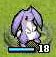# 星之一角

What have you found for these years?

## 2009-12-01

### powerset (3) hanoi

hanoi 的三個柱子，可以看成 source, buffer, 和 destination.

buffer, 然後把自己搬到 dest, 最後再把 buffer 裡的搬到 dest.

(n-1) source => buffer (buffer 與 dest 互換)
(n) source => destination (唯一真正的移動)
(n-1) buffer => destination (buffer 與 source 互換)

n 是盤子編號，f 是 from(source), b 是 buffer, t 是 to(dest),

`ha :: Integer -> Char -> Char -> Char -> [(Integer, Char, Char)] -> [(Integer, Char, Char)]ha 0 _ _ _ r = rha n f b t r = ha (n-1) f t b r ++ [(n, f, t)] ++ ha (n-1) b f t r`

`hanoi :: List a -> List a -> List a -> ...`

`ha' :: Integer -> Char -> Char -> Char -> [(Integer, Char, Char)]ha' 0 _ _ _ = []ha' n f b t = ha' (n-1) f t b ++ [(n, f, t)] ++ ha' (n-1) b f t`

`ha'' :: Integer -> Char -> Char -> Char -> [(Integer, Char, Char)]ha'' 0 _ _ _ = []ha'' n f b t = ha'' (n-1) f t b ++ ((n, f, t) : ha'' (n-1) b f t)`

ICFP 2009 演講全程錄影上網

#### 0 retries: